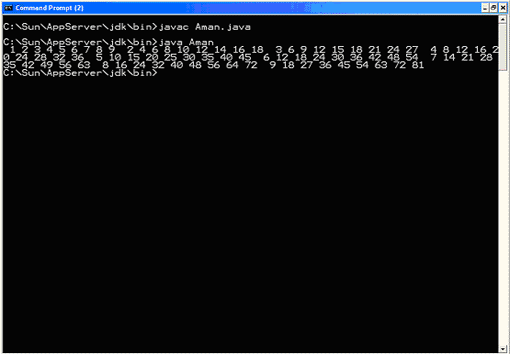## Core Java

Java Evolution
Overview of Java Language
Constant, Variable and Datatype
Operator and Expression
Decision making, Branching and Looping
Class Object and Method
Array and String
Inheritance
Package
Exception Handling
Applet
Managing Input/Output Files in Java
Examples of Core Java

# Decision making, Branching and Looping

### The for statement

 1) It is useful when the number of passes (iterations) can be predetermined The general syntax for (initialization; condition; update) { statements; } where: initialization - represents the declaration of one or more local variables of the same data type. When loop processing is complete, all such variables are destroyed. condition - represents a binary expression that, if true, allows the loop to continue. The condition is tested prior to each iteration. If no longer true, processing continues at the first statement after the closing brace of the for loop. update - represents one or more expressions to be executed at the end of each iteration. The braces may be omitted if the loop consists of a single statement. This would constitute a "single statement for loop".
 Example 1: Counting to 10 with a local variable for (int i = 1; i <= 10; i++) System.out.println(i); This is a single statement for loop. Local variable i is initialized to 1 before the first iteration and is used for loop control (its value determines when the loop will end). As long as i is less than or equal to 10, looping will continue. At the end of each iteration, i is incremented. Within each iteration, the current value of i is displayed. When the condition is no longer true, processing will continue at the next statement in the program and variable i will be destroyed.
 Example 2: Counting to 10 without a local variable Counting to 10 without a local variable int i = 1; for (; i <= 10; i++) System.out.println(i); System.out.println("Now i is " + i); Here loop control variable i is initialized outside the loop, it will not be destroyed when the loop completes. Its final value (which will be 11) is displayed by the statement after the loop. Notice that when no initialization expression is coded, a place holding semicolon is still required.
 Example 3: Coding multiple initialization and update expressions for (int i = 1, j = 10; i <= 10; i++, j--) System.out.println(i + " x " + j + " = " + (i * j)); This loop initializes two local variables, i and j, of the same data type before the first iteration. At the end of each iteration, i is incremented and j is decremented. Within each iteration, the product of i and j is displayed. Notice that commas are used to separate multiple initialization and update expressions. It can be nested. For example, the following program generates a simple 9 x 9 multiplication table: import java.io.*; public class Aman { public static void main(String[] args) { // This outer loop generates one row of the multiplication // table during each iteration. for (int row = 1; row <= 9; row++) { // This inner loop generates one column of the current row // of the multiplication table during each iteration. for (int column = 1; column <= 9; column++) { // If a one digit number is about to be displayed, preceed it // with four spaces. Otherwise, preceed it with three spaces. if ((row * column) < 10) { System.out.print(" "); } else { System.out.print(" "); } // Display the number. System.out.print((row * column)); } // End the current line. System.out.print(" "); } } } Output: